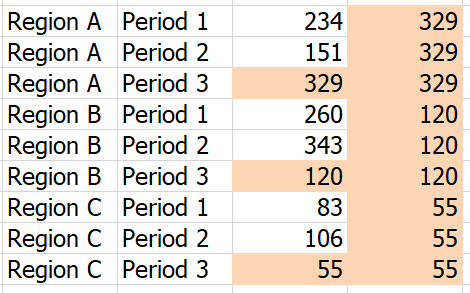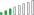cancel
Showing results for
Did you mean:Anonymous
Not applicable

## A column based on the last value in a different column

Hello,

I'm not sure how to create a column that always takes data from a different column, but only from the last period and duplicates it for all previous periods.

What I mean is something like the screenshot, third column is the one I want to create based on column with values1 ACCEPTED SOLUTIONCommunity Support

Hi @Anonymous

Create a column

`Column = CALCULATE(MAX(Sheet1[period]),ALLEXCEPT(Sheet1,Sheet1[region]))`

create a measure

`Measure = CALCULATE(MAX(Sheet1[target]),FILTER(ALLEXCEPT(Sheet1,Sheet1[region]),[period]=[Column]))`Best Regards

Maggie

Community Support Team _ Maggie Li
If this post helps, then please consider Accept it as the solution to help the other members find it more quickly.

5 REPLIES 5Resolver III

@Anonymous

Hey!

I typically use LOOKUPVALUE for situations like this.

Here is a link to documentation on that that particular function:

https://docs.microsoft.com/en-us/dax/lookupvalue-function-daxAnonymous
Not applicable

Thank you for your reply. I'm still struggling to make it work, it says that it doesn't support date type.

Also as I read the documentation I'm not sure if it woudn't leave other rows blank instead of duplicating the result (if it worked with date type)?

Should this give me the year end target column on the screen?

Year End Target = LOOKUPVALUE(Table[Target], Table[Period], "01.12.2018")Super User

Hi @Anonymous

It is not quite clear whether you want a measure in a table visual or a calculated column in your table.

A) For a measure (with Region, Period and Target in values of the table visual):

```Measure Year End Target =
VAR _LatestPeriod =
MAX ( Table1[Period] )
RETURN
CALCULATE ( DISTINCT(Table1[Target]), Table1[Period] = _LatestPeriod )```

B) For an additional calculated column in your table (assuming its columns are Region, Period and Target as you show):

```Measure Year End Target =
VAR _LatestPeriod =
CALCULATE ( MAX ( Table1[Period] ), ALLEXCEPT ( Table1, Table1[Region] ) )
RETURN
CALCULATE (
DISTINCT(Table1[Target]),
Table1[Period] = _LatestPeriod,
ALL ( Table1[Target] )
)```Anonymous
Not applicable

Hey @AlB, thank you for helping. I think that a calculated column would be better here, but regardless of which one I try to execure, I can't use my column Target as first argument in Calculate function.

CALCULATE (
Table1[Target],
Table1[Period] = _LatestPeriod,
ALL ( Table1[Target] )
)

What I have is a visual table, inside it there is Region, Actual, Target, Deviation, Percentage Deviation. Whole visual is filtered for just the current month. So there is only monthly data inside it.

Now I need a deviation from a year end target (so a deviation between eg. february actual and december target), but I don't know how to do this if visual is filtered for just the current month, thats why I wanted to duplicate a column with just values from the last period.Community Support

Hi @Anonymous

Create a column

`Column = CALCULATE(MAX(Sheet1[period]),ALLEXCEPT(Sheet1,Sheet1[region]))`

create a measure

`Measure = CALCULATE(MAX(Sheet1[target]),FILTER(ALLEXCEPT(Sheet1,Sheet1[region]),[period]=[Column]))`Best Regards

Maggie

Community Support Team _ Maggie Li
If this post helps, then please consider Accept it as the solution to help the other members find it more quickly.